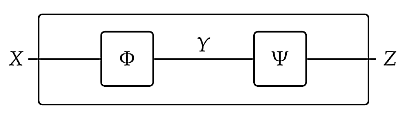Thanks for catching all those typos, Dan!

> 3. In puzzle 207, I think the feasibility relations compose the other way around, that is, it should have been \$$\Phi \Psi : \mathbb{N} \nrightarrow [0,\infty) \$$.

Ugh - yes! But since I'd just been talking about \$$\Psi\Phi\$$ in a general abstract situation, I want my example to be about a feasibility relation called \$$\Psi\Phi\$$ . So I'm going to switch the names \$$\Phi\$$ and \$$\Psi\$$ in puzzles 205 and 206.

Sorry! Things are already confusing enough, given that this feasibility relationis called \$$\Psi\Phi\$$. That's because when we apply first a function \$$f\$$ and then a function \$$g\$$, we usually call the composite \$$g f\$$ or \$$g \circ f\$$.